# Some Questions About Thevenin's Theorem

rtareen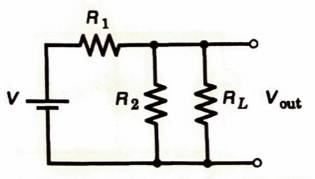(1) We want to find the voltage across ##R_L##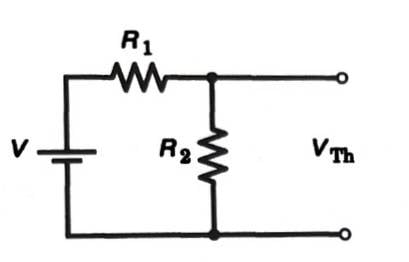(2) We remove the load and label the terminals ##V_T##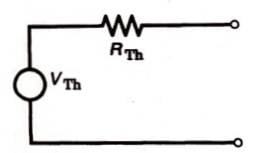(3) The equivalent network of (2)

So basically the voltage across the load is ##V_{th}## but when we find the equivalent network we put that same voltage behind a Thevenin resistance? Wouldn't that change the voltage across the load? Is the load in parallel with the Thevenin voltage?

Edited now to make the post prettier because you can't preview when posting in this forum.

Last edited:

Gold Member
First, thanks for an easy to read and understand question.

So basically the voltage across the load is Vth but when we find the equivalent network we put that same voltage behind a Thevenin resistance?
No. The Thevenin voltage is found after the load is removed. When you find the voltage on the output terminals with the load removed, you are taking advantage of the fact that in the Thevenin equivalent circuit, there is no current flowing through Rth when the load is removed. So, in that case, the output voltage is equal to the Thevenin voltage because there is no voltage drop across Rth.

Wouldn't that change the voltage across the load?
Yes, the voltage depends on the amount of resistance on the output terminals. The Thevenin voltage is defined as the voltage at the output with no load (∞Ω).

Is the load in parallel with the Thevenin voltage?
No. the load is in parallel with the series combination of the Thevenin voltage and the Thevenin resistance. Just as you have shown in the third schematic.

I guess you are supposed to use Thevenin's theorem to solve this. But, honestly, it's kind of a waste of effort. The whole circuit isn't any harder to solve than the circuit without the load resistor.

rtareen
First, thanks for an easy to read and understand question.
You're welcome =). I like to keep things neat and organized, because that's always more practical than putting in minimum effort and then waiting for the problems to pile up later. Prevention is better than cure. Sorry for the mini-rant.

No. The Thevenin voltage is found after the load is removed. When you find the voltage on the output terminals with the load removed, you are taking advantage of the fact that in the Thevenin equivalent circuit, there is no current flowing through Rth when the load is removed. So, in that case, the output voltage is equal to the Thevenin voltage because there is no voltage drop across Rth.

Yes, the voltage depends on the amount of resistance on the output terminals. The Thevenin voltage is defined as the voltage at the output with no load (∞Ω).

If the voltage across the output terminals is only equal to the Thevenin voltage when there is no current (or no load), then how it can it be used as an equivalent network when we try to reduce a more complex network? What I'm trying to ask is how the circuit can work the same given that the voltage across the load is not going to be the Thevenin voltage, yet when we solve for the Thevenin voltage we are essentially solving for the voltage across the open load terminals. But then, when we add the Thevenin resistance in there and reconnect the load, that voltage across the load is no longer the Thevenin voltage. Is there something I'm missing in my understanding?

I guess you are supposed to use Thevenin's theorem to solve this. But, honestly, it's kind of a waste of effort. The whole circuit isn't any harder to solve than the circuit without the load resistor.

We are just using this circuit as an introduction to Thevenin's theorem. I'm going through the book (Intro to Modern Electronics by Faissler) by myself so I don't have to suffer as much during the actual course next semester.

Staff Emeritus
What I'm trying to ask is how the circuit can work the same given that the voltage across the load is not going to be the Thevenin voltage, yet when we solve for the Thevenin voltage we are essentially solving for the voltage across the open load terminals.
I think you're getting confused by semantics. In your diagram 3, the voltage marked ##V_{th}##, I usually call ##V_{oc}## for open circuit. The voltage at the terminals, you marked ##V_{th}## in diagram 2. I like to call that terminal voltage. In any case, your two uses of ##V_{th}## are contradictory.

•DaveE
rtareen
I think you're getting confused by semantics. In your diagram 3, the voltage marked ##V_{th}##, I usually call ##V_{oc}## for open circuit. The voltage at the terminals, you marked ##V_{th}## in diagram 2. I like to call that terminal voltage. In any case, your two uses of ##V_{th}## are contradictory.
These are figures from the book. I didn't make these.

Staff Emeritus
These are figures from the book. I didn't make these.
OK, then the two uses in the book are contradictory. Errors in textbooks are not unheard of. Sometimes, they even print lists of errata for textbooks.

rtareen
I think you're getting confused by semantics. In your diagram 3, the voltage marked ##V_{th}##, I usually call ##V_{oc}## for open circuit. The voltage at the terminals, you marked ##V_{th}## in diagram 2. I like to call that terminal voltage. In any case, your two uses of ##V_{th}## are contradictory.
So you're saying the voltage of the open circuit terminals is different from the Thevenin voltage? Then what is the Thevenin voltage? Let's say I've computed the voltage across the open terminals ##V_{oc}##. How do you get ##V_{th}## so you can reduce the network like in Figure 3 of OP?

Last edited:
Gold Member
What I'm trying to ask is how the circuit can work the same given that the voltage across the load is not going to be the Thevenin voltage, yet when we solve for the Thevenin voltage we are essentially solving for the voltage across the open load terminals. But then, when we add the Thevenin resistance in there and reconnect the load, that voltage across the load is no longer the Thevenin voltage. Is there something I'm missing in my understanding?
I'm not sure that your really missing anything. It sounds like you are asking "How can Thevenin's Theorem be true?" It's a good question and one most beginning EE courses don't actually answer (i.e. prove). It is one of the really useful features of networks of linear components.

The proof for the simplest networks can be done by solving with KVL & KCL and comparing that solution to the solution of the Thevenin equivalent. For more complex networks, you'll want to learn about and use the superposition theorem to break the problem down into simpler parts.

A rigorous proof would be covered in a network theory class, which most EEs never actually take (I wish I didn't have to take the one I did, LOL). You can search for "proof of Thevenin's theorem" to find more detailed arguments, like this one:

rtareen
I'm not sure that your really missing anything. It sounds like you are asking "How can Thevenin's Theorem be true?" It's a good question and one most beginning EE courses don't actually answer (i.e. prove). It is one of the really useful features of networks of linear components.

The proof for the simplest networks can be done by solving with KVL & KCL and comparing that solution to the solution of the Thevenin equivalent. For more complex networks, you'll want to learn about and use the superposition theorem to break the problem down into simpler parts.

A rigorous proof would be covered in a network theory class, which most EEs never actually take. You can search for "proof of Thevenin's theorem" to find more detailed arguments, like this one:
I'm not a person who stresses about proofs for well-known and often-used theorems, however it is nice to know. I'm more asking "How does Thevenin's theorem make sense"? We're basically saying:

A voltage across a load is equal to the voltage across that load replaced with an open circuit minus some voltage given by the properties of the network its connected to.

Is that a valid summary I can put in my notes? You can add to it/change it if you want.

•Tom.G
Gold Member
I'm not a person who stresses about proofs for well-known and often-used theorems, however it is nice to know. I'm more asking "How does Thevenin's theorem make sense"? We're basically saying:

A voltage across a load is equal to the voltage across that load replaced with an open circuit minus some voltage given by the properties of the network its connected to.

Is that a valid summary I can put in my notes? You can add to it/change it if you want.
Yes. The Thevenin voltage is the obvious part, the real magic here is the equivalent resistance calculation; "the properties of the network its connected to". If you keep going with your analog electronics studies, you'll use this more (maybe). We would also call Rth "the driving point impedance" of the output port.

rtareen

•DaveE
Gold Member
So you're saying the voltage of the open circuit terminals is different from the Thevenin voltage? Then what is the Thevenin voltage? Let's say I've computed the voltage across the open terminals ##V_{oc}##. How do you get ##V_{th}## so you can reduce the network like in Figure 3 of OP?
I think what he's getting at is it is a different voltage because it's in a different location. But, in the no load case, it happens to have the same value as Vth in the Thevenin equivalent network.

He is absolutely correct IMO. You will find it very confusing if you mix up names and values when you are working with circuits. Naming things, like Voc, or Il, is somewhat trivial and arbitrary. Finding the values and/or relationships between them after that requires precision.

This is especially important when you are working with equivalent circuits or simplifications. In this case, the Thevenin equivalent circuit is a great simplification for how the circuit interacts with the load. But, in the process, you have lost information about what happens inside the network, for example the current through R1; this is the price to pay for simplification.

rtareen
I'm having another problem now. How do you find the equivalent resistance when we have a current source instead of a voltage source? I attached a screenshot of the problem (6-5) which uses Figure 6-18. We replace the current source with an open circuit, but the equivalent resistance should be ##R_{th} = \frac{500(1000)}{500 + 1000} = 333 \Omega##, right? Why are they saying it is 1000? Does it have to do with the fact there is an open circuit?

#### Attachments

• Thevenin.pdf
615.3 KB · Views: 86
Gold Member
I'm having another problem now. How do you find the equivalent resistance when we have a current source instead of a voltage source? I attached a screenshot of the problem (6-5) which uses Figure 6-18. We replace the current source with an open circuit, but the equivalent resistance should be ##R_{th} = \frac{500(1000)}{500 + 1000} = 333 \Omega##, right? Why are they saying it is 1000? Does it have to do with the fact there is an open circuit?
After you have replaced the current source with an open circuit, what is the current through the 500Ω resistor? Where would that current flow? Draw the schematic with the current source removed.

rtareen
After you have replaced the current source with an open circuit, what is the current through the 500Ω resistor? Where would that current flow? Draw the schematic with the current source removed.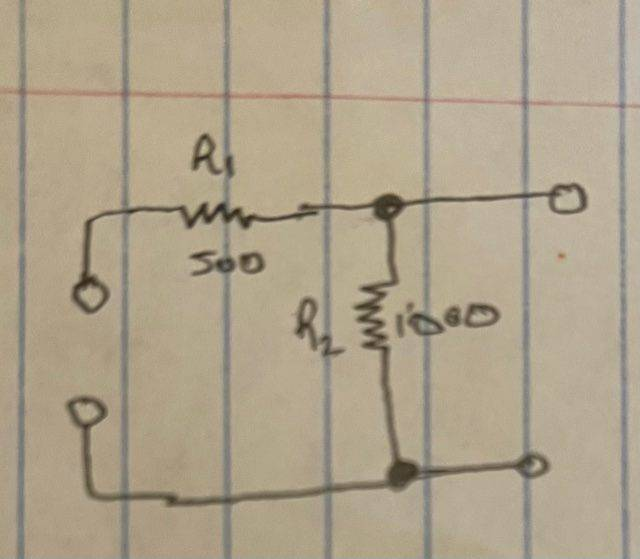Here's my drawing with the current source removed. Without the current source there is no current throughout the entire thing. However, the only thing I can think of to justify the answer is that if current were coming from the load, then that current would only go through R2.

Gold Member
View attachment 281585

Here's my drawing with the current source removed. Without the current source there is no current throughout the entire thing. However, the only thing I can think of to justify the answer is that if current were coming from the load, then that current would only go through R2.
Yes. Current can only flow through R2. So R1 can have no effect on the circuit. You would get the same answers if R1=500Ω, 1KΩ, or 100KΩ, so you can remove R1 also. You could also think of finding the total resistance of the circuit branch with R1, it's in series with ∞Ω from the current source you removed, so 500Ω + ∞Ω = ∞Ω.

When you find Rth by calculating the resistance seen from the output port, you are really, conceptually, applying a voltage to the output port and finding how much current would flow. For example, think of measuring the resistance with an ohm-meter; that is exactly what those meters do. That ohm-meter will only see current flowing through R2.

rtareen
Hi DaveE.

Thanks a lot for your help. I'm actually self-studying right now from the book. Can you check my answer to problem #2? It seems to simple for this to be the right answer (these are my latex notes). This should be my last question on this topic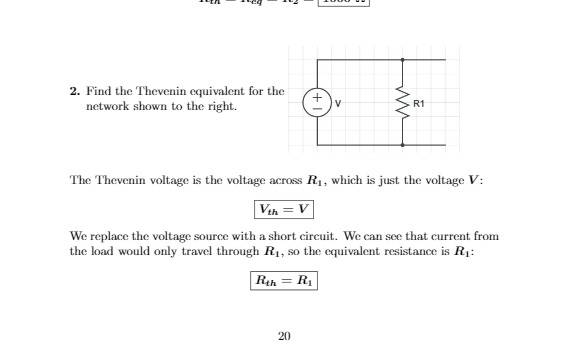Gold Member
Hi DaveE.

Thanks a lot for your help. I'm actually self-studying right now from the book. Can you check my answer to problem #2? It seems to simple for this to be the right answer (these are my latex notes). This should be my last question on this topic

View attachment 281587

I personally think that one is incorrect.

Just for the sake of checking if I were to put ##R_L## there, then the voltage across ##R_L## would be ##V## and the current going through it would be ##V/R_L##.

If I were to say that ##V_{th} = V## (which I agree there) and ##R_{th}=R_1##, then what would happen if I replaced that circuit with the "Thevenin equivalent" circuit and attach it to ##R_L##? Now the voltage across ##R_L## would be a voltage divider and the current going through it is ##V/(R_1+R_L)##. That doesn't look right to me.

I think ##R_{th}=0##. If ##R_{th}=0##, then when I replace that circuit with the Thevenin equivalent and connect ##R_L## the voltage across would be ##V## and the current going through it would be ##V/R_L##. That's what I was expecting. Sometimes I struggle with the kind of inspection method to get ##R_{th}##; so: I sometimes short circuit the output and get the current going through that, then I say that ##R_{th}=V_{oc}/I_{sc}##. The reason I got ##R_{th}=0## is because when I try this approach I get ##I_{sc}=\infty## and ##R_{th}=V/\infty = 0##.

edit:

Funny enough... in their description... if you replace ##V## with a short circuit, then none of the current would want to travel through ##R_1## because it would all travel through the short circuit ##0 \Omega## wire.

rtareen
I personally think that one is incorrect.

Just for the sake of checking if I were to put ##R_L## there, then the voltage across ##R_L## would be ##V## and the current going through it would be ##V/R_L##.

If I were to say that ##V_{th} = V## (which I agree there) and ##R_{th}=R_1##, then what would happen if I replaced that circuit with the "Thevenin equivalent" circuit and attach it to ##R_L##? Now the voltage across ##R_L## would be a voltage divider and the current going through it is ##V/(R_1+R_L)##. That doesn't look right to me.

I think ##R_{th}=0##. If ##R_{th}=0##, then when I replace that circuit with the Thevenin equivalent and connect ##R_L## the voltage across would be ##V## and the current going through it would be ##V/R_L##. That's what I was expecting. Sometimes I struggle with the kind of inspection method to get ##R_{th}##; so: I sometimes short circuit the output and get the current going through that, then I say that ##R_{th}=V_{oc}/I_{sc}##. The reason I got ##R_{th}=0## is because when I try this approach I get ##I_{sc}=\infty## and ##R_{th}=V/\infty = 0##.

edit:

Funny enough... in their description... if you replace ##V## with a short circuit, then none of the current would want to travel through ##R_1## because it would all travel through the short circuit ##0 \Omega## wire.
Will that method always work? ##R_{th} = V_{oc}/I_{sc}##? I need a standard way to always do this kind of problem.

By the way, the book says that the Thevenin resistance is the resistance "looking back into the output terminals". But they never explained what that means. This is a really old book that only had one edition but for some reason my university likes to use it for this electronics course.

Last edited:
Gold Member
That's how my professor taught it to me and it has been working for me :)

rtareen
That's how my professor taught it to me and it has been working for me :)
cool !

rtareen
I just realized what you are doing is using the relation ##V_{th} = R_{nor}I_{nor}##. Thats a relation given in my book! Really cool I can't wait for an exam question about this.

Gold Member
@rtareen
The other general rule to remember:
Just as an Ideal current source has Infinite resistance, an Ideal voltage source has Zero resistance.

Note the use of 'Ideal'. In the real world of course nothing is really ideal, but it is a good place to start for that 'First Look'!

Cheers,
Tom (and have fun!)

Gold Member
Will that method always work? Rth=Voc/Isc?
Yes

Gold Member
Will that method always work? Rth=Voc/Isc?
Let's just assume that Thevenin's theorem is correct. Then whatever network you have can be represented by the 3rd schematic in the OP; a Voltage source Vth in series with an impedance Rth.

Then what is the open circuit voltage, Voc? Since there is no current flow there is no voltage across the resistor so Voc = Vth.

What is the short circuit current, Isc? All of the voltage is across Rth, so it must be Isc = Vth/Rth = Voc/Rth. Thus Rth = Voc/Isc.

Both of these measurements are made at the output terminals, so it doesn't really matter what is "inside" the network connected to those terminals, as long as it is made of linear components (i.e. Thevenin's theorem is valid).

Staff Emeritus
I wish the textbooks would teach it the simple way. You have a black box with two terminals. You don't know what's inside the box, but you're told it is linear.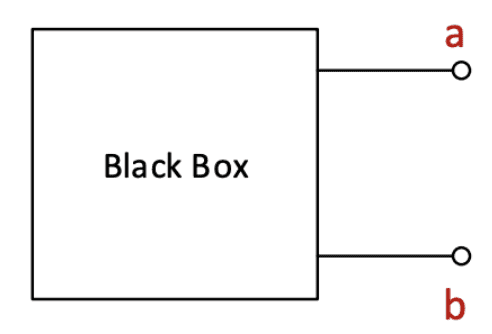So what simple and universal experiments could you do using only the terminals? Measure open circuit voltage VOC and measure short circuit current ISC. Then draw a straight line between them (because it's linear).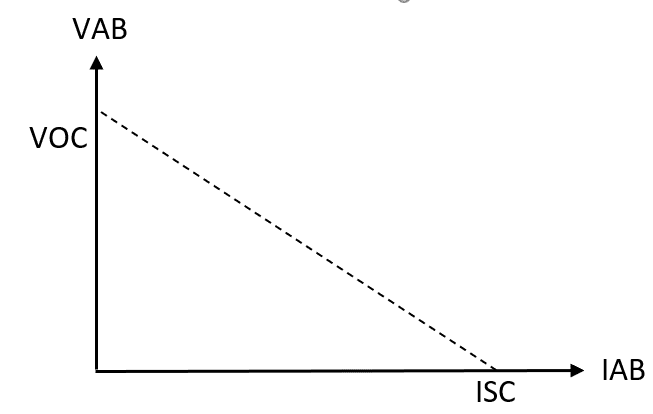The simple circuit with voltage VOC in series with resistance ##\frac{VOC}{ISC}## draws the same straight line, and is therefore equivalent. There can be other equivalent circuits, but this is the one we call Thevenin's Equivalent.

There is no need to define any other confusing quantities, VTH, ITH, RTH.

Thevenin's Theorem takes more math to prove that this simple procedure is always valid. But the theorem should not be confused with the simple procedure to find the equivalent.

In power grid analysis, I've made equivalents like that to represent Canada, in studies of the US power grid.

•DaveE